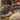August 19, 2019

# Part 4

## Chapter 8 - Booleans

I diverged from laracasts here. I didn’t want to make multiple \<li> tags, so I used a conditional which is in the next chapter. The code is as follows:

`// index.view.php<!-- Above HTML omitted for brevity --><ul>  <?php foreach (\$task as \$key => \$value) : ?>    <li>      <strong><?= ucwords(\$key); ?>: </strong>        <?php if (\$key == 'completed') {          \$value = (\$value) ? 'Completed' : 'Incomplete';        }; ?>      <?= \$value; ?>    </li>  <?php endforeach; ?></ul><!-- Below HTML omitted for brevity -->`

Yes, I know it looks like a lot but lets highlight the keypart:

`<?php if (\$key == 'completed') {  \$value = (\$value) ? 'Completed' : 'Incomplete';}; ?>`
Line 1:
`<?php if (\\$key == 'completed')`

If the current key equal to the string ‘completed’, then move to the next line, if it’s not equal to ‘completed’, then move past the curly braces.

Line 2: `\$value = (\$value) ? 'Completed' : 'Incomplete';`
set \$value equal to 'Completed' if TRUE set \$value equal to 'Incomplete' if FALSE 'Incomplete'
This is called the "ternary operator" should you want to read more
Line 3: `}; ?\>`
End the block, continue to the endNote:
I cannot find any definitive documentation as to whether to use:
`True vs TRUE vs true`
`False vs FALSE vs false`
All of the above are booleans.

This suggests to use `true` and `false` for booleans

## Chapter 9 - Conditionals

Plain if statement in php:

`<?phpif (condition) {  do stuff} else {  do other stuff};`

If statements that drop down to plain html to make it more readable:

`<!-- more HTML --><?php if (condition) : ?>  <p><strong>Words</strong></p><?php else : ?>  <h1>Do other stuff</h1><?php endif; ?><!-- more HTML -->`

Checking if something is NOT true:

`<?php\$boolean = trueif (! \$boolean){  // Will run if the value of \$boolean == false} else {  // Will run if the value of \$boolean == true}`

This is called the “BANG” operator.

This is all I got for chapter 9. Lets move to chapter 10.